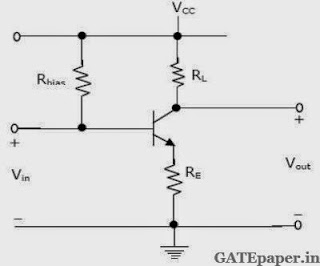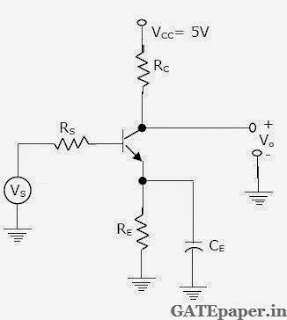### GATE 1997 ECE Analog Circuits (Analog Electronics) - Video Solutions

1. In the BJT amplifier shown in figure, the transistor is based in the forward active region. Putting a capacitor across RE willa. Decrease the voltage gain and decrease the input impedance
b. Increase the voltage gain and decrease the input impedance
c. decrease the voltage gain and increase the input impedance
d. Increase the voltage gain and increase the input impedance

2. A cascode amplifier stage is equivalent to
a. A common emitter stage followed by a common base stage
b. A common base stage followed by an emitter follower
c. An emitter follower stage followed by a common base stage
d. A common base stage followed by a common emitter stage

3. The output voltage Vo of the circuit shown isa. – 4 volts
b. 6 volts
c. 5 volts
d. – 5.5 volts

4. A half wave rectifier uses a diode with a forward resistance Rf. The voltage is Vmsinωt and the load resistance is RL. The DC current is given by5. Negative feedback in
1. Voltage Series configuration
2. Current Shunt configuration
a. Increases input impedance
b. Decreases input impedance
c. Increases closed loop gain
Solution:

6. The transistor in the circuit shown is so biased (dc biasing network is not shown) that the dc collector current, IC = 1 mA and VCC = 5 volts. The network components have following values: RC = 2 kΩ, RS = 1.4 kΩ and RE = 100 Ω. The transistor has β = 100 and a base spreading resistance, rbb’ = 100Ω. Assume VT = 25 mV.Evaluate small signal voltage gain AVS at a frequency of 10 kHz, and input resistance Ri for two cases:
a. CE, the bypass capacitor across RE is 25 µF
b. The bypass capacitor CE is removed leaving RE unbypassed

7. Consider the circuit given in the figure is using an ideal operational amplifier. The characteristics of the diode are given by the relation I = IS(eqV/KT – 1), where V is the forward voltage across the diode.a. Express Vo as function of Vi , assuming Vi > 0
b. If R = 100 kΩ, IS = 1 µA and VT = 25 mV, find the input voltage Vi for which Vo = 0.

8. In circuit shown, assume that the operational amplifier is ideal and that Vo = 0 volts initially. The switch is connected first to ‘A’ charging C1 to the voltage V. it is then connected to the point ‘B’. This process is repeated 'f' times per second.a. Calculate the charge transferred per second from node A to node B.
b. Derive the average rate of change of the output voltage Vo.
c. If the capacitor and the switch are removed and a resistor is connected between points A and B, find the value of the resistor to get the same average rate of change of the output voltage?
d. If the repetition rate of the switching action is 104 times per second, C1 = 100pF, C2 = 10pF and V= 10 mV. What is the average change of the output voltage?

9. An IC 555 chip has been used to construct a pulse generator. Typical pin connections with components are shown below. It is desired to generate a square pulse of 10 kHz.Evaluate values of RA and RB if the capacitor C has the value of 0.01 µF for the configuration chosen. If necessary you can suggest modification in the external configuration.